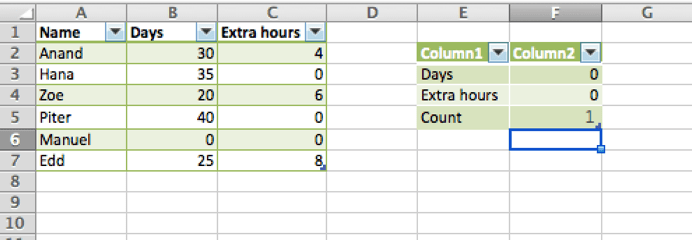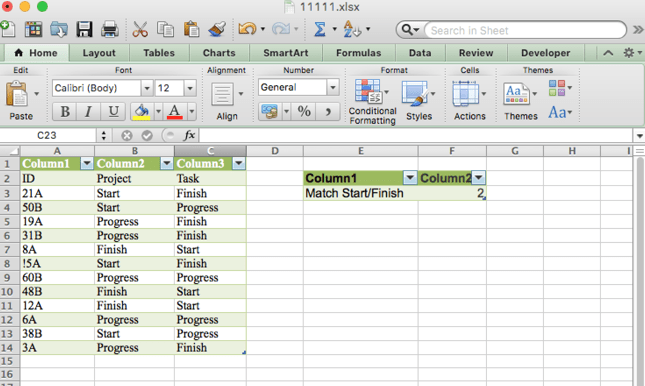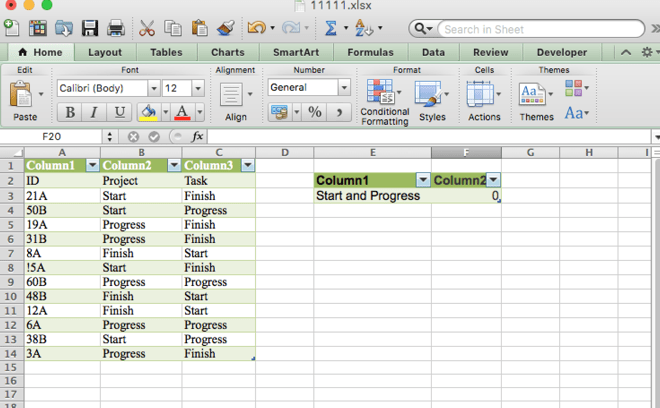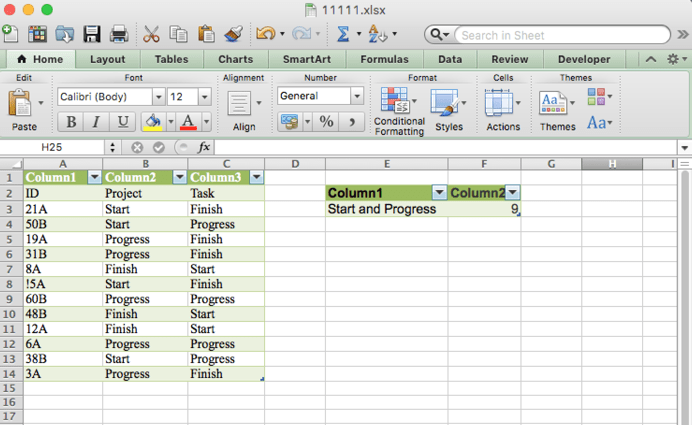Get instant live expert help with Excel or Google Sheets“My Excelchat expert helped me in less than 20 minutes, saving me what would have been 5 hours of work!”

#### Post your problem and you’ll get expert help in seconds.

Your message must be at least 40 characters
Our professional experts are available now. Your privacy is guaranteed.

# How to Use COUNTIF With Multiple Criteria in Excel

You use the COUNTIF function in Excel when you want to count cells based on a single criterion. If you want to count based on multiple criteria, you will use the COUNTIFS function. Here is how to make the best use of this function.

## Using COUNTIF with multiple criteria

Syntax for a single criteria is :

`=COUNTIF(range, criteria)`

The best function you can use for situations when you need to count cells based on multiple criteria is the COUNTIFS function. The “s” on the end of Countif makes it plural, and that means that there are multiple criteria.

You can use up to XX criteria_range and criteria pairs in a single COUNTIFS function, each criterion ranges one MUST be the same shape.

You should note that the COUNTIFS function takes all of the criteria_range/criteria pairs and counts only those instances where all of the pairs are true.

### COUNTIFS formula with the same criteria

If you want to count items with identical criteria, you still need to supply each criteria_range / criteria pair individually.

For example, here is the right formula to count items that have 0 both in column B and column C:

`=COUNTIFS(\$B\$2:\$B\$7,"=0", \$C\$2:\$C\$7,"=0")`

This COUNTIFS formula returns 1 because only “Manuel” have “0” value in both columns.If you use simple formula with a single criteria_range like COUNTIFS(B2:C7,”=0″) would show a different result – the total count of cells in the range B2:C7 containing a zero (which is 4 in this example).

### COUNTIFS with two criteria in different criteria ranges

When you understand how to use COUNTIFS with one critera_range and criteria, then it becomes easy to extend it to two or more criteria_range/criteria combinations.

For example, let’s count the number of times the combination of Start and Finish appear in our spreadsheet :

`=COUNTIFS(B3:B14,"Start",C3:C14,"Finish")`

As you can see, this formula has returned the correct number of rows where both criteria are matched in their respective columns.

It’s worth repeating one of the rules of COUNTIFS here – the two criteria ranges must be the same shape and size or your formula won’t work, and will return a #VALUE error. Here’s an example where the second criteria range is smaller than the first:

`=COUNTIFS(B2:B13,"Start",C2:C12,"Finish")`### COUNTIFS with two criteria in the same criteria range

Another way to use COUNTIFS is to use the same criteria with more than one criteria_range. For example, suppose we want to count the number of times either Start or Progress appears in the Product column. You can try this formula using COUNTIFS:

`=COUNTIFS(B2:B13,"Start",B2:B13,"In Progress")`

Unfortunately, this formula will return a value of 0, as shown here:### Common errors with COUNTIFS

This is a common mistake that even experienced Excel users make when using COUNTIFS (and its counterpart, SUMIFS which you can learn about here).

The problem is that COUNTIFS looks in our criteria_range, B2:B13 and tries to count cells that contain both Start and Progress. Remember that we wanted to count cells that contain either Start or Progress. Of course, there are no cells in our range that contain both Start and Progress, so the result is 0.

Ironically, the best way to find the answer in this scenario is so simple that it is often overlooked – just use two COUNTIF functions in your formula, rather than once COUNTIFS function, like this:

`=COUNTIFS(B2:B13,"Start")+COUNTIF(B2:B13,"Progress")`

In other words, we simply count all the rows that contain Start, and add those to the tally of rows that contain Progress.Still need some help with Excel formatting or have other questions about Excel? Connect with a live Excel expert here for some 1 on 1 help. Your first session is always free.

### Did this post not answer your question? Get a solution from connecting with the expert.Another blog reader asked this question today on Excelchat:
Related blogs
Solution examplesI have a spreadsheet that contains a list of names/IDs and I want to generate a formula that inserts into a cell the number of times a name or ID appears on that list. Either insert a column into that spreadsheet or transfer that info into a column on a different spreadsheet. Either way would be fine.
Solved by O. L. in 18 minshow can i turn my sumifs into a countifs because my sumifs works great and i also want to count the thing im summing
Solved by O. C. in 40 minsHello, I have a spreadsheet with 3159 rows. One column consists of 9-digit numbers in each of the 3159 rows. I need the formula that can calculate how many of those numbers appear more than once.
Solved by D. H. in 56 minsI need a formula to count the number of rows that contain at least one of two possible phrases, but not give me the total number of instances that both phrases occur overall.
Solved by E. B. in 33 minsI need a formula that has these 2 rules. If letter was rec'd between 1st and 15th = 1st of the month following letter rec'd date. If letter was rec'd between 16thst and end of the month= 1st of the 2nd month following letter rec'd date.
Solved by V. F. in 20 mins## Subscribe to Excelchat.coAnother blog reader asked this question today on Excelchat: